# Math > Year 2

## Measuring & Comparing Capacity in litres & ml

Capacity
Capacity is defined as the maximum quantity of something an object can hold.

Units of capacity
Capacity can be measured in litres and millilitres.

Litre is the standard unit of capacity.A litre is a bigger unit and a millilitre is a smaller unit of capacity.

How can we measure the capacity of the container?

We use measuring jugs to know the capacity of the containers.

Example: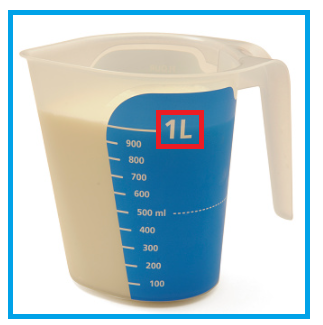The jug can hold a liquid of 1l or 1,000 ml. Hence, the measuring jug has a capacity of 1l.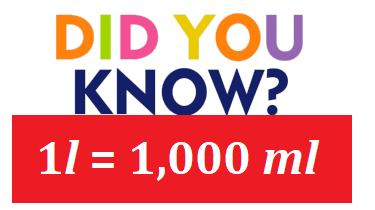How can we compare the capacities?

We use three symbols to compare.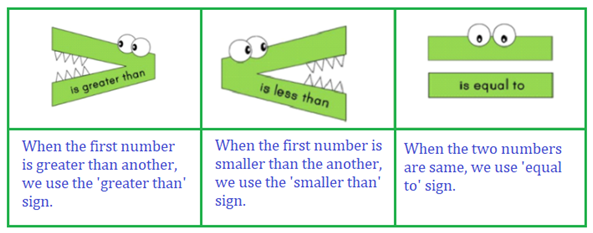Let’s learn with the examples:

Choose the correct symbol.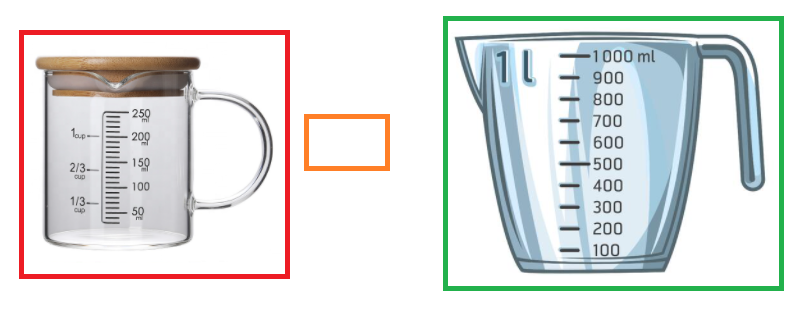250 ml < 1,000ml

250 ml is less than 1,000 ml.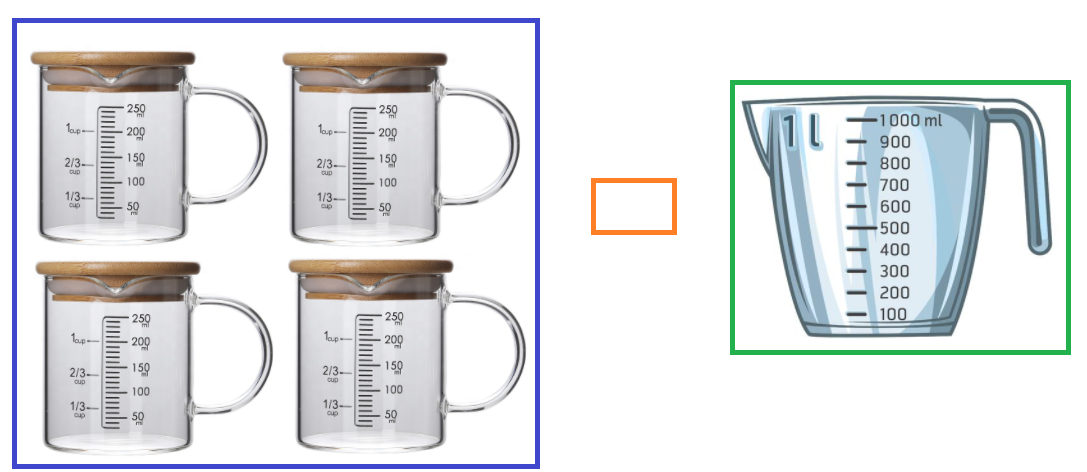250 ml + 250 ml + 250 ml + 250 ml = 1,000ml

1,000 ml = 1,000 ml

4 cups of 250 ml are equivalent to 1 jug of 1,000 ml.

Let’s try a question:

Question: Which container has more capacity?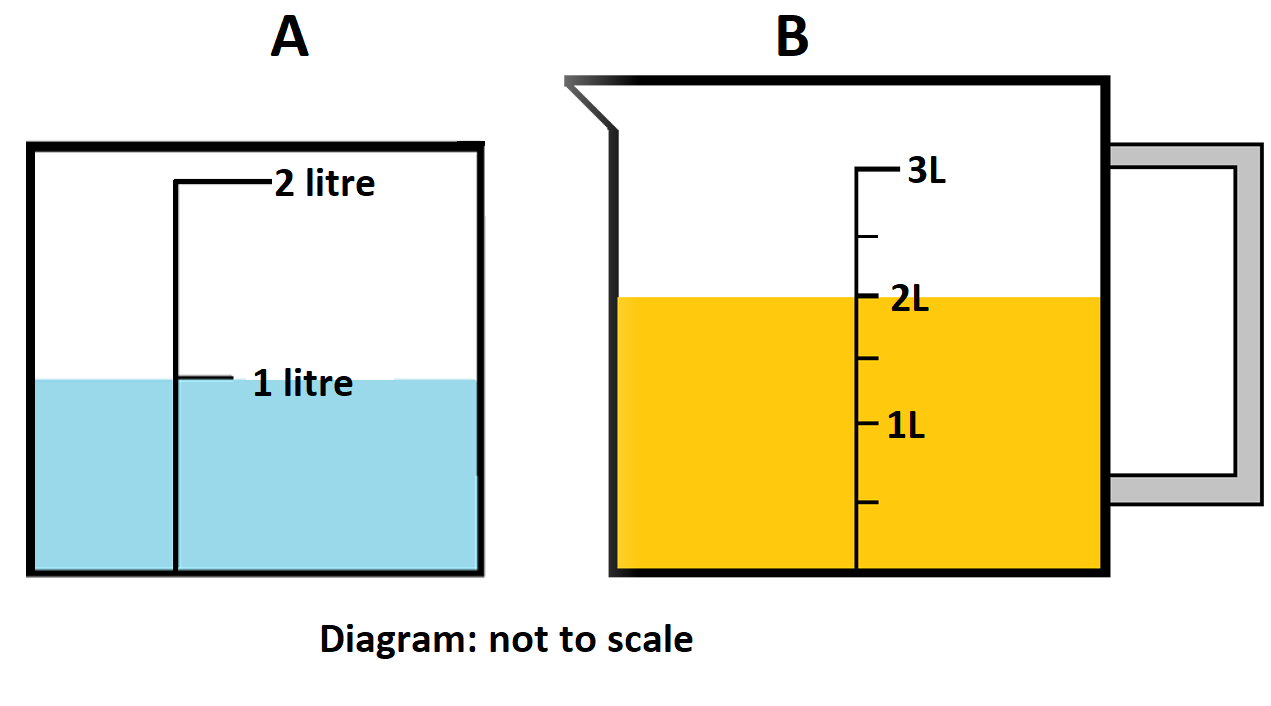Explanation:

The capacity of the container A = 2l

The capacity of the container B = 3l

The capacity of container B is more than container A.

//